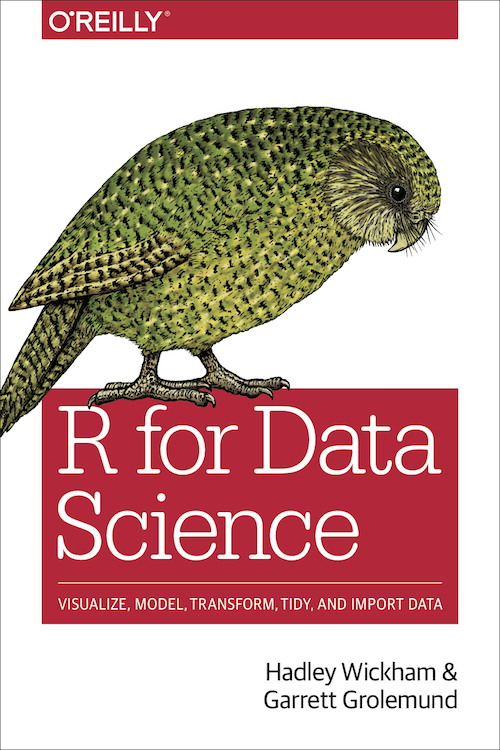# R for Data: Learn How to Use R

A research guide on using R to work with data.

## Open Textbooks on R

### R for Data Science: Visualize, Model, Transform, Tidy, and Import Data by Hadley Wickham & Garrett Grolemund (2017)

An introductory text that will help you learn the most important tools in R and how they are utilized in data science, including the following common steps in data science projects:

• Importing data into R
• Tidying, transforming, and wrangling the data
• Statistical analysis and modeling of the data
• Visualizing the data with a variety of graphs### R for Social Sciences by Data Carpentry

An introduction to basic concepts, skills, and tools for working with data in R, aimed at social sciences researchers. The content is organized into hands-on lessons, and instructor notes are provided.

Topics covered:

• Overview of the RStudio interface
• Basic R syntax and data types
• Importing CSV files into R
• Working with data frames
• Calculating summary statistics from a data frame
• Data wrangling, including selecting, adding, or removing rows and columns
• Data visualization, including scatterplots, box plots, and bar plots
• R Markdown, which integrates R code with text and plots